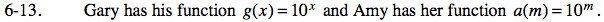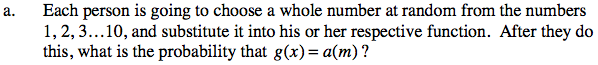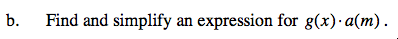### Home > A2C > Chapter 6 > Lesson 6.1.1 > Problem6-13

6-13.
1. Gary has his function g(x) = 10x and Amy has her function a(m) = 10m . Homework Help ✎

1. Each person is going to choose a whole number at random from the numbers 1, 2, 3…10, and substitute it into his or her respective function. After they do this, what is the probability that g(x) = a(m)?

2. Find and simplify an expression for g(x).a(m).Notice that g(x) and a(m) are equivalent functions, what is the probability that Gary and Amy pick the same number?

Assume Gary has chosen a number. How many chances does Amy have to pick the same number?
Out of how many choices?Remember the exponent rules.

10x + m